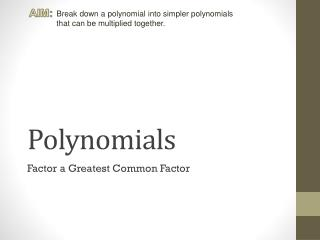DownloadDownload PresentationPolynomials

# Polynomials

Télécharger la présentation## Polynomials

- - - - - - - - - - - - - - - - - - - - - - - - - - - E N D - - - - - - - - - - - - - - - - - - - - - - - - - - -
##### Presentation Transcript

1. AIM: Break down a polynomial into simpler polynomials that can be multiplied together. Polynomials Factor a Greatest Common Factor

2. AIM: Break down a polynomial into simpler polynomials that can be multiplied together. Factorization Tree What are the factors of 4x2y? 4x2y 4  x2 y  2   2 x y  x  The factorization is 22xxy.

3. AIM: Break down a polynomial into simpler polynomials that can be multiplied together. GCF What is the GCF of 4 and 8? 1 2 Birthday Cake 4 4 8 The GCF of 4 & 8 is 4.

4. AIM: Break down a polynomial into simpler polynomials that can be multiplied together. GCF of Polynomials What is the GCF of 4x2y and 8x? 1xy +2 x 1x2y +2x 4 4x2y +8x The GCF is 4x. The factors are 4x(xy + 2)

5. AIM: Break down a polynomial into simpler polynomials that can be multiplied together. Check your answer You can check your answer by multiplying …. 4x(xy + 2) 4x2y 4x2y + 8x + 8x That’s what you were asked to factor!

6. AIM: Break down a polynomial into simpler polynomials that can be multiplied together. Factor a Cylinder! SAcylinder = 2πr2 + 2πrh r +h r r2 +rh r2 +rh πr2 +πrh πr2 +πrh π 2 2πr2 +2πrh The GCF is2πr. The factors are 2πr(r + h)

7. AIM: Break down a polynomial into simpler polynomials that can be multiplied together. Factor a Trinomial Factor 3s3 + 6s2 – 3s s2 +2s –1 s3 +2s2 –s s3 +2s2 –s s 3 3s3 +6s2 –3s The GCF is 3s. The factors are 3s(s2 + 2s – 1)